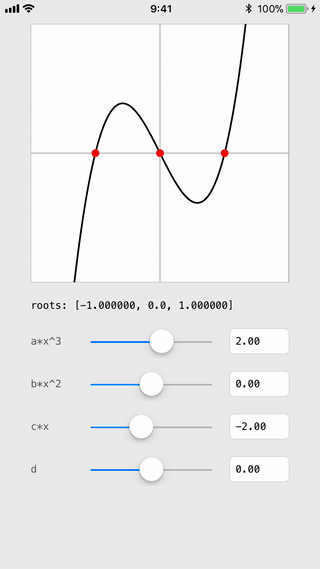Swiftpack.co - Package - dnpp73/CubicEquationSolver

# CubicEquationSolver## What is this

Cubic Equation Solver written in Swift.

I tested only iOS 10 - 12. maybe works fine macOS too.

``````// find roots of `ax^3 + bx^2 + cx + d = 0`
// returns only real number
func solveCubicEquation(a: Double, b: Double, c: Double, d: Double) -> [Double]
``````

## For What?

I had to find `y` for any `x` in the cubic Bézier curve `B(t) = (x, y)`.

so I had to convert them to cubic equations `y = f(x)` and solve...

## Carthage

https://github.com/Carthage/Carthage

Write your `Cartfile`

``````github "dnpp73/CubicEquationSolver"
``````

and run

``````carthage bootstrap --no-use-binaries --platform iOS
``````

MIT